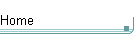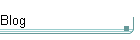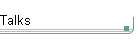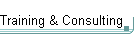GotW #36On theblogNovember 4: Other Concurrency Sessions at PDC November 3 October 26: Hoare on Testing October 23This is the original GotW problem and solution substantially as posted to Usenet. See the book More Exceptional C++ (Addison-Wesley, 2002) for the most current solution to this GotW issue. The solutions in the book have been revised and expanded since their initial appearance in GotW. The book versions also incorporate corrections, new material, and conformance to the final ANSI/ISO C++ standard.

## InitializationDifficulty: 3 / 10

What's the difference between direct initialization and copy initialization, and when are they used?### Problem

#### JG Question

1. What is the difference between "direct initialization" and "copy initialization"?

(Hint: See an earlier GotW.)

#### Guru Question

2. Which of the following cases use direct initialization, and which use copy initialization?

```  struct T : S {
T() : S(1),             // base initialization
x(2) {}           // member initialization
X x;
};

T f( T t ) {              // passing a function argument
return t;               // returning a value
}

S s;
T t;
S& r = t;
reinterpret_cast<S>(t);   // performing a reinterpret_cast
static_cast<S>(t);        // performing a static_cast
dynamic_cast<T&>(r);      // performing a dynamic_cast
const_cast<const T&>(t);  // performing a const_cast

try {
throw T();              // throwing an exception
} catch( T t ) {          // handling an exception
}

f( T(s) );                // functional-notation type conversion
S a = { 1, 2, 3 };     // brace-enclosed initializers
S* p = new S(4);          // new expression```### Solution

1. What is the difference between "direct initialization" and "copy initialization"?

(Hint: See an earlier GotW.)

Direct initialization means the object is initialized using a single (possibly conversion) constructor, and is equivalent to the form "T t(u);":

```    U u;
T t1(u); // calls T::T( U& ) or similar```

Copy initialization means the object is initialized using the copy constructor, after first calling a user-defined conversion if necessary, and is equivalent to the form "T t = u;":

```    T t2 = t1;  // same type: calls T::T( T& ) or similar
T t3 = u;   // different type: calls T::T( T(u) )
//  or T::T( u.operator T() ) or similar```

[Aside: The reason for the "or similar"s above is that the copy and conversion constructors could take something slightly different from a plain reference (the reference could be const or volatile or both), and the user-defined conversion constructor or operator could additionally take or return an object rather than a reference.]

NOTE: In the last case ("T t3 = u;") the compiler could call both the user-defined conversion (to create a temporary object) and the T copy constructor (to construct t3 from the temporary), or it could choose to elide the temporary and construct t3 directly from u (which would end up being equivalent to "T t3(u);"). Since July 1997 and in the final draft standard, the compiler's latitude to elide temporary objects has been restricted, but it is still allowed for this optimization and for the return value optimization. For more details, see GotW #1 (about the basics) and GotW #27 (about the 1997 change).

#### Guru Question

2. Which of the following cases use direct initialization, and which use copy initialization?

Section 8.5 [dcl.init] covers most of these. There were also three tricks that actually don't involve initialization at all... did you catch them?

```  struct T : S {
T() : S(1),             // base initialization
x(2) {}           // member initialization
X x;
};```

Base and member initialization both use direct initialization.

```  T f( T t ) {              // passing a function argument
return t;               // returning a value
}```

Passing and returning values both use copy initialization.

```  S s;
T t;
S& r = t;
reinterpret_cast<S>(t);   // performing a reinterpret_cast```

Trick: A reinterpret_cast does no initialization of a new object at all, but just causes t's bits to be reinterpreted (in-place) as an S.

`  static_cast<S>(t);        // performing a static_cast`

A static_cast uses direct initialization.

```  dynamic_cast<T&>(r);      // performing a dynamic_cast
const_cast<const T&>(t);  // performing a const_cast```

More tricks: No initialization of a new object is involved in either of these cases.

```  try {
throw T();              // throwing an exception
} catch( T t ) {          // handling an exception
}```

Throwing and catching an exception object both use copy initialization.

Note that in this particular code there are two copies, for a total of three T objects: A copy of the thrown object is made at the throw site, and in this case a second copy is made because the handler catches the thrown object by value.

`  f( T(s) );                // functional-notation type conversion`

"Constructor syntax" type conversion uses direct initialization.

`  S a = { 1, 2, 3 };     // brace-enclosed initializers`

Brace-enclosed initializers use copy initialization.

`  S* p = new S(4);          // new expression`

New-expressions use direct initialization.